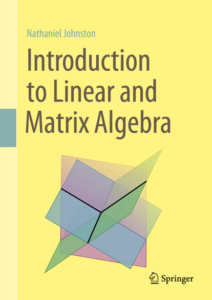## Introduction to Linear and Matrix AlgebraThis textbook emphasizes the interplay between algebra and geometry to motivate the study of linear algebra. Matrices and linear transformations are presented as two sides of the same coin, with their connection motivating inquiry throughout the book. By focusing on this interface, the author offers a conceptual appreciation of the mathematics that is at the heart of further theory and applications. Those continuing to a second course in linear algebra will appreciate the companion volume Advanced Linear and Matrix Algebra.

Starting with an introduction to vectors, matrices, and linear transformations, the book focuses on building a geometric intuition of what these tools represent. Linear systems offer a powerful application of the ideas seen so far, and lead onto the introduction of subspaces, linear independence, bases, and rank. Investigation then focuses on the algebraic properties of matrices that illuminate the geometry of the linear transformations that they represent. Determinants, eigenvalues, and eigenvectors all benefit from this geometric viewpoint. Throughout, “Extra Topic” sections augment the core content with a wide range of ideas and applications, from linear programming, to power iteration and linear recurrence relations. Exercises of all levels accompany each section, including many designed to be tackled using computer software.

Introduction to Linear and Matrix Algebra is ideal for an introductory proof-based linear algebra course. The engaging color presentation and frequent marginal notes showcase the author’s visual approach. Students are assumed to have completed one or two university-level mathematics courses, though calculus is not an explicit requirement. Instructors will appreciate the ample opportunities to choose topics that align with the needs of each classroom, and the online homework sets that are available through WeBWorK.

### Citation/bibliographic information:

• Author: Nathaniel Johnston
• Cite as: N. Johnston. Introduction to Linear and Matrix Algebra. Springer International Publishing, 2021.
• DOI: 10.1007/978-3-030-52811-9
• eBook ISBN: 978-3-030-52811-9
• Hardcover ISBN: 978-3-030-52810-2
• BibTeX entry:
```@book{johnston2021introduction,
title={Introduction to Linear and Matrix Algebra},
author={Nathaniel Johnston},
isbn={978-3-030-52810-2},
url={https://www.springer.com/us/book/9783030528102},
year={2021},
publisher={Springer International Publishing}
}```